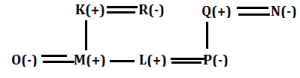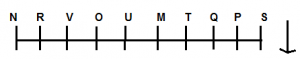Latest Banking jobs   »   Reasoning Ability Quiz For IBPS Clerk...

# Reasoning Ability Quiz For IBPS Clerk Prelims 2021- 3rd August

Directions (1-3): Study the information carefully and answer the questions given below.
Seven persons i.e. P, Q, R, S, T, U and V are scoring different marks in an examination. P score more marks than R. T score more marks than V and less than U. P does not score the highest. S score less than T and more than only one person. R score more than U.

Q1. Who among the following person score highest marks?
(a) U
(b) R
(c) Q
(d) T
(e) None of these

Q2. How many persons score less than V?
(a) One
(b) None
(c) Two
(d) Three
(e) Five

Q3. Who among the following person score third highest?
(a) V
(b) P
(c) R
(d) T
(e) None of these

Q4. Anchal is 30th from the left end of a row and Teena is 21st from the right end of row. If they interchanged their positions then anchal ranks become 32 from left end. Find total number of persons in the row?
(a) 51
(b) 47
(c) 58
(d) 52
(e) None of these

Q5. Demon’s ranked 24th from the top in a class of 64 students. What is the rank of demon from the bottom?
(a) 40
(b) 32
(c) 41
(d) 29
(e) None of these

Direction (6-8): Study the following information carefully and answer the question below-
Eight persons K, L, M, N, O, P, Q and R are from two generations and four married couple. Q and N are married couples K is father in law O who is sister in law of L. L and P are married couples. P is daughter in law of R. M and L are siblings of same gender. N is mother of P.

Q6. How is P related to M?
(a) Son-in-law
(b) Son
(c) Sister -in-law
(d) Daughter
(e) None of these

Q7. If J is the only child of L then how is N related to J?
(a) Maternal Grand mother
(b) Mother
(c) Aunt
(d) Father
(e) None of these

Q8. How is L related to K?
(a) Son
(b) Son in law
(c) Brother -in-law
(d) Father
(e) None of these

Directions (9-11): Study the following information carefully and answer the question given below-
There are ten people i.e. M, N, O, P, Q, R, S, T, U and V who have seated on a bench, faces towards South direction. M and T sits together but both of them do not sit at any extreme end. There a 4 people sit between Q and V. Only two people sits left to Q. O sits immediate right of U. P sits 2nd left of T and S is the neighbor of P. There is three people between U and N who does not sit at left end of the bench.

Q9. Who among the following sits 2nd left of Q?
(a) R
(b) M
(c) S
(d) N
(e) None of these

Q10. How many people sit between U and M?
(a) Four
(b) Three
(c) Two
(d) One
(e) No one

Q11. Who among the following sits at extreme right end?
(a) P
(b) N
(c) S
(d) T
(e) V

Q12. If all the letters in the word “Reasonable” are arranged in alphabetical order from left to right in such a way that all vowels are arranged first followed by consonants, then how many letters are there in between O and S after the arrangement?
(a) Two
(b) One
(c) None
(d) Three
(e) Four

Q13. If in the number 639429687, 1 is added to each of the digit which is less than five and 1 is subtracted from each of the digit which is greater than five then how many digits are repeating in the number thus formed?
(a) Two
(b) One
(c) None
(d) Three
(e) Four

Q14. Which of the following elements should come in a place ‘?’ ?
DF8 HJ12 LN16 ?
(a) PR19
(b) PR18
(c) PR21
(d) PR22
(e) None of these

Q15. How many pairs of letters are there in the word ‘Perspective’, each of which have as many letters between then in the word as they have between then in the English alphabet?
(a) Two
(b) One
(c) Four
(d) More than Four
(e) Three

Solutions

Solutions (1-3):
Sol. Q>P>R>U>T>S>V
S1.Ans(c)
S2.Ans(b)
S3.Ans(c)

S4. Ans(d)
Sol. Total number of persons in the row=(21+32-1)=52

S5. Ans(c)

Solutions (6-8):
Sol.S6. Ans. (c)
S7. Ans. (a)
S8. Ans. (a)

Solutions (9-11):
Sol.S9.Ans.(c)
S10.Ans.(e)
S11.Ans.(b)

S12. Ans(e)
Sol. After arrangement: AAEEOBLNRS

S13. Ans(a)

S14. Ans(e)
Sol. PR20

S15. Ans(d)
Sol. PR, PS, RS, PR, TV